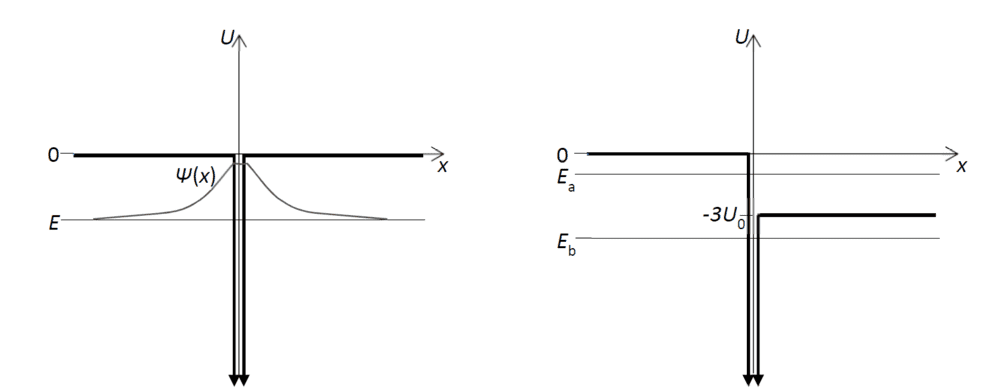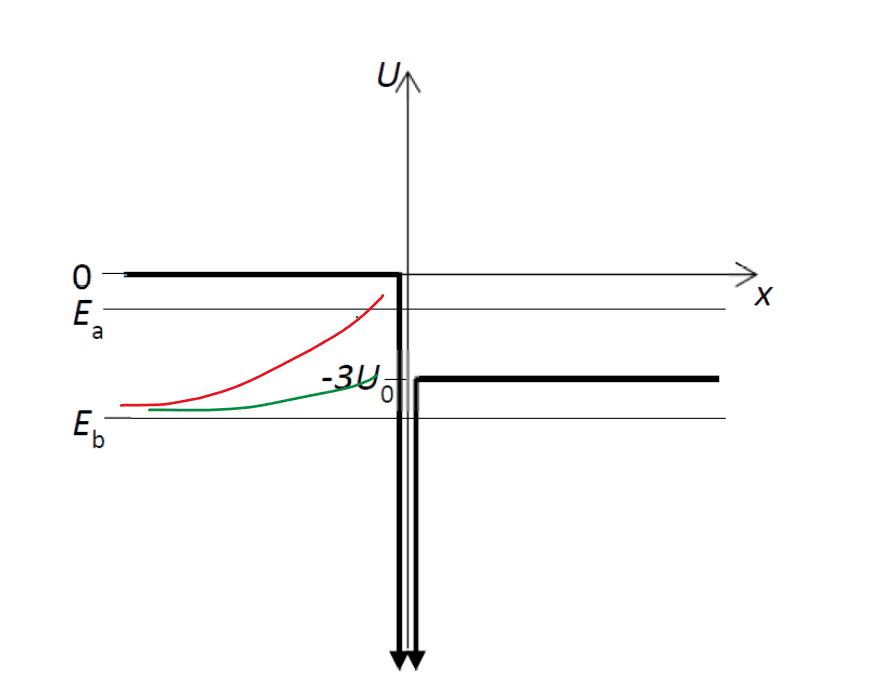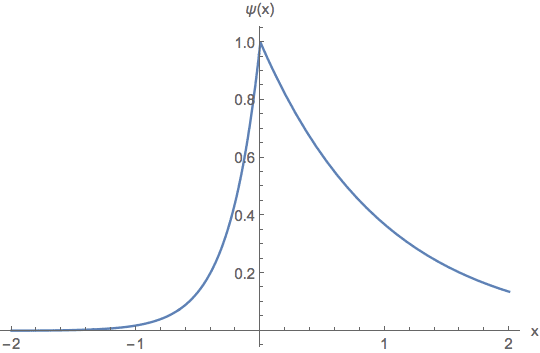# Semi-infinite potential well

Homework Statement:
How to approach questions with
Relevant Equations:
$$−ℏ^2/2m d^2ψ(x)/dx2 +U(x)ψx=Eψ(x)$$
Hello folks,

So my level of quantum knowledge is equivalent to what is covered in (year one) two short chapters introducing the topic in Knight's Physics for Scientists and Engineers. Ch. 39 introduces the idea of a wavefunction in a pretty simple way, and ch. 40 touches provides the basics of 1D QM looking at a particle in a box and the essence of infinite square potential wells.

I am looking to apply those basics to the scenario below. The example below comprises an infinitely deep well which is narrow such that in the left hand case, the wave function does not significantly change across its width. I am trying to understand how I would sketch the wave function for the two energy positions Ea and Eb for the right-hand well and work out the Schrodinger equation outside x<0 and x>0, giving the general solution?I can answer this sort of question for the straightforward case of an infinite square potential well as it's covered in the textbook and there is a lot of good coverage online. But I'm not familiar with how to extend that case to this one? For example, why, if the left-hand case has infinitely deep walls, does the wave function extend beyond the walls?

Are there any accessible resources (first year physics friendly) that will help me with this? Or any explanation that members here might be able to share.

kuruman
Homework Helper
Gold Member
Check out this link about the delta function potential. It should be accessible.

•StillAnotherDave
Many thank @kuruman I'll def check that.

kuruman
Homework Helper
Gold Member
Many thank @kuruman I'll def check that.

@kuruman I've used the link you posted to work out how to derive the wave function for a standard delta potential well.

But I appreciate now that the right hand example is of a delta potential combined with a potential step. How would this affect the wave function equation? Would the value of k be different on the right hand side?

E.g. for the left side: $$k=\frac{\sqrt{2mE}}{ℏ^2}$$

But for the right: $$k'=\frac{\sqrt{2m\left(E-3U\right)}}{ℏ^2}$$

Also, how would you determine the factor by which it is more probable for a particle to be say at -4U at x>0 compared to x<0?

Last edited:
Any and all help appreciated...

kuruman
Homework Helper
Gold Member
Any and all help appreciated...
I am sure you need a quick answer, but you have to realize that I am not tied to my computer 60/60/24/7. I just saw your post #5 and I am thinking how best to reply. Please don't follow a post with another post unless you receive some kind of answer first.

•etotheipi
kuruman
Homework Helper
Gold Member
In all such problems you have to decide whether you are looking for bound states or scattering states. The Wikipedia link has two sections in which these are discussed. For the symmetric potential, when E>0, you have scattering states whether the particles are incident from the right or from the left; when E<0, you have a single bound state for the single delta function potential.

Your asymmetric potential is a bit more complicated. For ##E>0##, you have scattering states whether the particles come in from the left or from the right, just like before; for ##−3U_0<E<0## you have scattering states for particles incident only from the right; for ##E<−3U_0##, you have bound states. So it seems to me that you need to specify which of the two scattering cases you are considering when you ask about probabilities.

Your analysis that you need two different ##k##'s is correct except that $$k'=\frac{\sqrt{2m\left(E-U\right)}}{ℏ^2}=\frac{\sqrt{2m\left(E+3U_0\right)}}{ℏ^2}.$$

Thanks. Before getting into the points you've brought up can I get clarity on two preliminary points.

[My questions all assume the energy level Eb on the diagram, therefore a bound state on both sides]

1. Sketching the wave function for the right-side diagram given energy level Eb: given there is a continuity condition, would the exponential curve for x<0 go to -3U or to 0? Because the curve for x>0 would be an exponential beginning at -3U.

2. If the energy level on the right is dropped to -3U does this affect how how the TISE is written for x>0. Is it, for example:

$$\frac{ℏ^2}{2m}\frac{d^2\psi }{dx^2}+U_{\left(x\right)}\psi _{\left(x\right)}=-4U_0$$

Regarding your response about the solution for the wave function. Given the two different values k and k' would it be:

$$Ae^{kx}+Ae^{-k'x}$$

And lastly, on the point about probabilities. The particle is assumed to be at -4U so the question about probability on the right vs left is assuming a bound state on both sides - the third option of the three you mentioned.

Last edited:
kuruman
Homework Helper
Gold Member
In that case you have two different wavefunction, one for the left and one for the right.
##\psi_L(x)=N e^{k_Lx}## and
##\psi_R(x)=N e^{-k_Rx}##
where ##N## is the normalization constant that you have to find by doing two integrals, one from negative infinity to zero and one from zero to infinity. The ratio of these integrals should be the factor you need.

Last edited:
Is it correct that the wave function on the left is an exponential which goes to ##E=-3U_0## on the left instead of ##0##? In order to be continuous? Or would it still go to zero on the left?

My intuition is that in the former case, the value for ##k## would be the same on both sides given both functions are constrained by the lower energy value. But if the function still goes to zero on the left then you get two different values for ##k## on either side.

In which case, it should go to zero on the left as we discussed above that there are different ##k## values... but would the function be continuous in that case?

kuruman
Homework Helper
Gold Member
Is it correct that the wave function on the left is an exponential which goes to ##E=-3U_0## on the left instead of ##0##? In order to be continuous? Or would it still go to zero on the left?
I don't understand your question. What does "goes to ##E=-3U_0## on the left instead of ##0##" mean? If you are asking about the asymptotic behavior, both wavefunctions must go to zero very far from the origin. The line at ##U=0## on the negative ##x##-axis and the line at ##U=-3U_0## along the positive ##x##-axis are not asymptotes, if that's what you're thinking. They represent the potential ##U(x)##.

I'm not confident with the precise language I should be using. But descriptively, what would the sketch of the wave function look like in the case that there is a step. Does the curve on the left (x<0) still go upto ##U=0##?which is correct for x<0?

kuruman
Homework Helper
Gold Member
I'm not confident with the precise language I should be using. But descriptively, what would the sketch of the wave function look like in the case that there is a step. Does the curve on the left (x<0) still go upto ##U=0##?
Descriptively, the unnormalized wavefunctions are
##\psi_L(x)=e^{k_Lx}## and ##\psi_R(x)=e^{-k_Rx}##. Pick different numerical values for ##k_L## and ##k_R## and plot the first one for ##x<0## and the second one for ##x>0##. They are both equal to unity at the origin and vanish very far from the origin. The one with the large ##k## drops to zero faster. What else is there in a decaying exponential?

It is late where I am and I have to get some sleep.

Last edited:
I'm gonna take that to mean red.

kuruman
Homework Helper
Gold Member
I'm gonna take that to mean red.
Whatever "red" means, take it at face value. Like most people I need to sleep, especially when it's way past my usual bedtime.

DrClaude
Mentor
I'm not confident with the precise language I should be using. But descriptively, what would the sketch of the wave function look like in the case that there is a step. Does the curve on the left (x<0) still go upto ##U=0##?
The y axis has units of energy, nothing to do with the wave function. You can scale it as you wish.

kuruman